• ## LED亮度调节原理

万次阅读 2018-08-15 23:54:21
近年来Light-emitting diode（LED）被广泛用于照明行业，相较于其他光源，LED具有诸多优势：光效高、寿命长、色温变化小、动态响应快、体积小等。那么如何才能设计出合理的灯具呢，我来给大家罗列一下LED的主要特性...

近年来Light-emitting diode（LED）被广泛用于照明行业，相较于其他光源，LED具有诸多优势：光效高、寿命长、色温变化小、动态响应快、体积小等。那么如何才能设计出合理的灯具呢，我来给大家罗列一下LED的主要特性。

一. 发光原理

当电流被注入到半导体的PN结时，原子中低能级的电子吸收能量从基态被激发到较高能级，这个能级我们称之为激发态。而激发态的寿命是短暂的，他十分容易回归到基态。在回归基态时激发态电子会释放出多余的能量，这些能量中有一部分以光子的形式传递了出去。这一过程称为能级跃迁。这里描述的是自发辐射的过程，有别于激光二极管的受激辐射。自发辐射产生的光子，光谱较宽，方向性较差，响应较慢，属于非相干光源。适用于低速光通信和普通的照明。

节能是各行各业不断努力的目标，“按需照明”需要根据环境的变化，人员的变化自动调节灯光的亮度，实现即不影响人的生产活动，又做到高效节能的目的。那么LED是怎么实现亮度调节的呢？

二.亮度调节

1.调节驱动电流图一是1w（额定电流为350mA）白光LED的驱动电流与发光强度对比图。可以看到，当电流为350mA时，光强为1倍，当175mA时，光强为0.5倍，在低于额定电流时基本保持线性。因此，通过调节电流的大小可以很好的控制LED的发光强度。

2.控制做功时间

在搬弄技术之前，先来提一个生理特征：当人看到一幅画面快速闪过时，这幅画面产生的视觉刺激会在大脑中停留几十到几百毫秒时间，亮度越亮，停留的时间越长。这一特征我们称为视觉残留
早在宋朝的时候，人们就已经利用这一生理现象发明了“走马灯”，到后来又出现了一帧一帧画出来的动画片，一格格播放的露天电影，都是利用了视觉残留这以特征。现在电影的标准是每秒播放24帧，也就是1秒钟只放24幅图片，而这在我们看来是非常连续，非常真实的。

a.直流DC

PWM（脉冲宽度调制）,这是一种应用非常广泛的调节方式，不仅可以用在亮度调节上，还用在逆变器，模型里的舵机，多轴飞行器里姿态控制都离不开PWM。如图二，如果我们要把一个20mA的LED灯的亮度调节到25%，我们可以把电流直接调到5mA，也可以让LED以20mA的电流亮25%的时间，灭75%的时间，如此循环，当这个循环足够的快，快到人眼便无法感到闪烁。这种调节工作时间（调节脉冲宽度）的方式就是PWM。
我们再套用公式来罗列一下上面的观点：
因为 W=P∗t=U∗I∗t$W=P\ast t=U\ast I\ast t$$W = P*t = U*I*t$
所以 0.25∗W=U∗(I∗0.25)∗t=U∗I∗(t∗0.25)$0.25\ast W=U\ast \left(I\ast 0.25\right)\ast t=U\ast I\ast \left(t\ast 0.25\right)$$0.25*W = U*(I*0.25)*t = U*I*(t*0.25)$
（由于LED在调节电流的过程中，正向导通电压变化不大，为了简化计数我们假设电压是不变的）

b.交流AC

电网的电都是以交流的形式传输到终端设备，可控硅是一种可以被控制什么时候被导通的半导体器件，即控制导通角，它会在电流0位置时自动关闭。所以可以通过可控硅直接控制输入到设备的电能。下图中咖啡色的线就是电网电压，蓝色线为被可控50%切相后的波形。c.各种调光方式的优缺点

真正的不闪式调光方式就是用通过调节电流来实现的。高频的电流脉冲可以使用小体积的电感就能轻而易举的滤成平顺的直流。所以好的护眼灯都应该是用这种方案的。再来说说电流调节的缺点吧，图一指出的电流与光强的关系并不是单纯的线性关系；而且更为微妙的是：图四中随着电流的减小，LED的光谱波长会变长，即红移（白光灯珠则表现为色温变暖）。虽然人对色温的偏差并不是太敏感，但是对色彩的差异还是非常敏感的，所以当需要精确控制亮度时（RGB混色）就比较麻烦了，需要通过算法来修正误差。

PWM调光的优点是系统简单，特别是需要做多路调光的时候。另外由于工作时的电流一直都是以额定电流工作，所以不存在调节电流的光强线性度与光谱偏移的问题。

切相调光是为了兼容白织灯时代所遗留的调光器。优点是，调光器存量市场巨大，特别是在美国。其实这种调光器一点都不适合LED灯具。白织灯因为是钨丝发热产生光，具有热惰性，钨丝不会应为电压被切掉而马上冷却停止发光。但是LED却是高动态的发光器件，要避免闪烁需要持续供电，为了在市电50/60Hz下不发生闪烁，需要在电路上做较多的处理，因此支持可控硅调光的LED灯的PF都做不高。
展开全文• 　前级功放的专业叫法是：前置放大器也是整套器材中对音色影响最大的部分，它是提供合适的音频电平信号，调节音质的器材(俗称前置放大器，接在音源和功率放大器之间)。　后级功放的专业叫法是：纯后级功放这才是真正...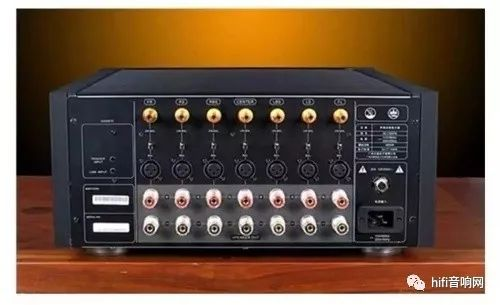关于前级和后级功放的区别前级和后级功放的区别——都是功放，作用却不同！　　前级功放的专业叫法是：前置放大器 也是整套器材中对音色影响最大的部分 ，它是提供合适的音频电平信号，调节音质的器材(俗称前置放大器，接在音源和功率放大器之间)。　　后级功放的专业叫法是：纯后级功放 这才是真正的功放部分，它对动态和低频控制力方面影响大。是单纯地把”前级“音频信号进行放大，以提供足够的功率驱动音箱喇叭发声的器材(总是接在音箱之前)。　　而一般家庭中常见的功放应该叫做前后级合并式放大机才对，合并功放。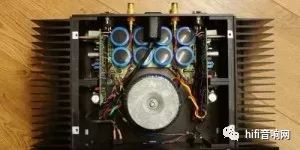后级功放(纯后级功放)：　　后级的输入讯号很单纯，就是承接前级的输出。但后级的负载是喇叭，这就是让许多音响迷，甚至杂志评论写手搞不定之处。后级是前级的负载，是高阻抗负载;喇叭是后级的负载，是低阻抗负载。看起来差不多，只差一个字，但阻抗的一高一低却造成很容易推或推不动现象。当前级接上高阻抗的后级，它主要提供适切的输出电压，因为后级扩大机。　　纯后级功放需要前置放大器来推动， 纯后级功放功率一般很大都在几百瓦以上，通常用在一些专业场所，并且多台同时工作推动不同的音箱，由于它被本身不带前置放大电路所以也就没有低电平输入端口，没有话筒等高阻输入信号的插口，这就需要在他前面加前置放大器或调音台给信号来控制它。 前置放大器和纯后级功放也有合二为一的，通常功率不大300瓦以下，应用于比较小一点的场所，家庭使用或KTV等使用。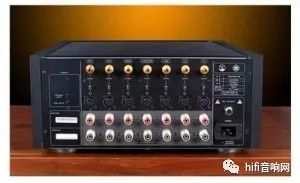前级功放(前置放大器)：　　前置放大器是指置于信源与放大器级之间的电路或电子设备，是专为接受来自信源的微弱电压信号而设计的。　　前置放大器用来放大弱信号的，一般都是先将信号通过电解电容来滤掉高频的噪音信号，然后进入负反馈的运放来放大信号。功率放大器一般指放大交流信号的功率 就是信号不失真的情况下放大用电器的电流和电压。前放紧靠探测器，传输线短，分布电容Cs减小,提高了信噪比。信号经前放初步放大，减少外界干扰的相对影响。前放设计为高输入阻抗,低输出阻抗实现阻抗转换和匹配。　　如果单论技术的话，前级比后级要求更精细，更难做好，如果要加特别的电源线的话，也绝对不能因为后级电流大而把好的线用在后级，应该是前级。　　前置放大器一般是连接纯后级功放的，前级输出接后级的输入端，后级接音箱就可以。前置放大器是放大电压，纯后级功放是放大电流，前置放大器是各种音源设备和功率放大器之间的链接设备，音源设备的输出信号电平都比较低，不能推动功率放大器正常工作，而前置放大器正是起到信号放大的作用。　　总结：　　功放一般分为前级功放、后级功放和合并级功放，合并机把前级、后级集于一身的机器。前级是用来把信号作初步放大、调节音量的；而后级则是把前级来的信号作大量放大来推动扬声器。关于前级和后级功放的区别http://www.zghifi.com/thread-22368-1-1.html(出处: HIFI音响)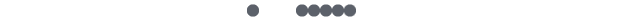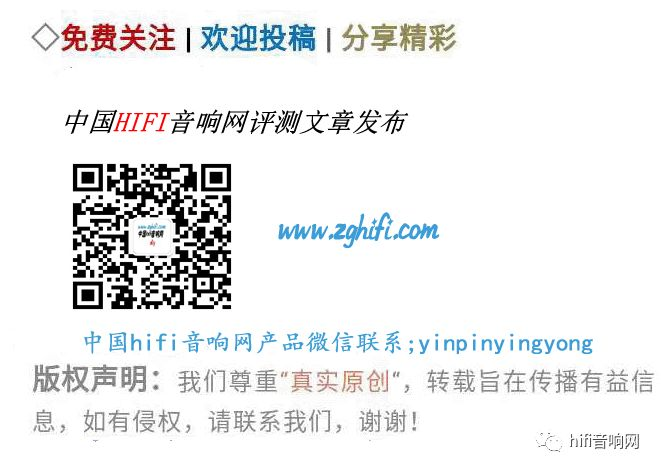展开全文• pyqt5+opencv 颜色阈值动态调节工具 两年前受1Zlab的代码启发，但直到最近需要使用了才想着用QT实现和优化一下。原本是想将图片都通过QImage模块显示的，但是QT似乎不支持HSV以及HLS颜色空间的显示，只好用OpenCV了...
pyqt5+opencv 颜色阈值动态调节工具
两年前受1Zlab的代码启发，但直到最近需要使用了才想着用QT实现和优化一下。原本是想将图片都通过QImage模块显示的，但是QT似乎不支持HSV以及HLS颜色空间的显示，只好用OpenCV了。
有个坑，不知道为什么调用OpenCV的inRange方法时会崩溃，也没有报错。无奈只好根据inRange方法的原理自己写了。等于阈值的情况我没有深入研究是否应该赋值255（实际上我赋值255），经过和inRange方法的测试发现效果似乎一致。
工具仅支持HSV以及HLS颜色空间。展开全文opencv python pyqt5 hsv
• 如何实现电源模块动态电压调节 如何实现电源模块动态电压调节 1. 简要介绍 2. 名词术语 3. 参考资料 4. 基础原理 5. 实例分析 5.1. 某有源反馈网络实现动态电压调整 5.1.1. 反馈网络设计 5.1.2. 反馈网络计算 ...


如何实现电源模块动态电压调节

如何实现电源模块动态电压调节

1. 简要介绍
2. 名词术语
3. 参考资料
4. 基础原理
5. 实例分析

5.1. 某有源反馈网络实现动态电压调整

5.1.1. 反馈网络设计
5.1.2. 反馈网络计算

5.2. RK3399/VDD_LOG 动态电压调整

5.2.1. 反馈网络设计
5.2.2. 反馈网络计算
5.2.3. 驱动配置修改

1. 简要介绍
处理器工作在全速状态下和空闲状态下所需要的电压是不同的, 动态电压调节（DVS）基于对系统的负载预测, 在一个开环电压控制系统中用多组不同能耗级别的频率、电压对来实现调节。如果使用常规的DC/DC变换器或线性变换器实现DVS, 可在电路上增加一些额外的元器件进行反馈, 使其向电源变换器反馈网络注入一小电流, 改变反馈比, 同时软件可以控制注入电流的大小及方向, 达到动态电压调整或自适应电压调节。
2. 名词术语

DVS: Dynamic Voltage Scaling, 动态电压调整

3. 参考资料

DVS与AVS的原理及设计应用
Dynamically Program Voltage Regulators
How to Dynamically Adjust Power Module Output Voltage
Methods of output-voltage adjustment for DC/DC converters

4. 基础原理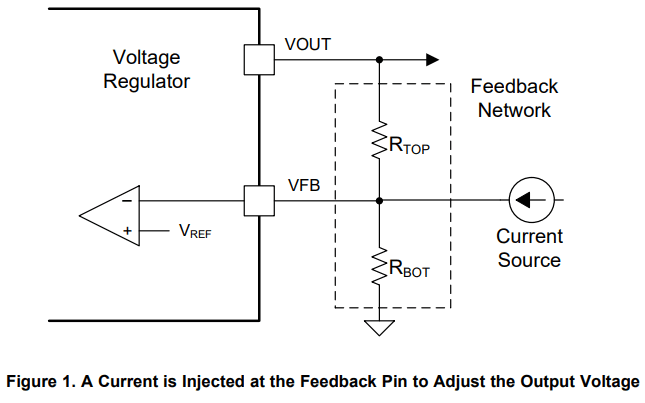当注入电流为正时, 流过$R_{BOT}$的电流增加, 相当于$R_{TOP}$阻值降低了, 故会引起输出电压降低。
当注入电流为负时, 流过$R_{BOT}$的电流减少, 相当于$R_{TOP}$阻值提高了, 故会引起输出电压升高。
5. 实例分析
5.1. 某有源反馈网络实现动态电压调整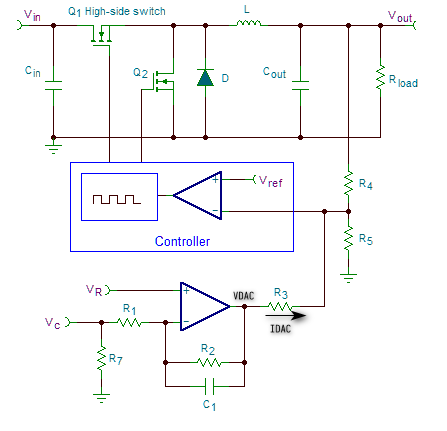5.1.1. 反馈网络设计
设流过 R3 进入 DC/DC 变换器的电流为 $I_{DAC}$, R3 左端的电压为 $V_{DAC}$, 则有
$I_{DAC}=\frac{V_{DAC}-V_{REF}}{R3}$
$V_{OUT}=V_{REF}(1+\frac{R4}{R5}) - I_{DAC}R4$
$\frac{V_{C}-V_{R}}{R1}=\frac{V_{R}-V_{DAC}}{R2}$
经整理可得
$V_{OUT}=V_{REF}(1+R4(\frac{R3+R5}{R3R5})) + \frac{R4}{R3}(V_{C}\frac{R2}{R1} - V_{R}\frac{R1+R2}{R1})$
同时
$F_{PWM} > \frac{10}{R2C1}$
5.1.2. 反馈网络计算

假设选用的变换器的 $V_{REF}=1.21V$, 运放的工作电压为5V, 经过电阻分压提供给其正向输入端作为参考电压 $V_{R}=2.5V$
运放工作模式为电压跟随器, 设 $R1=R2=10K$, 则 $V_{DAC}=5-V_{C}$
假设控制电压范围为 $V_{C}=[0, 5V]$, 输出电压调整范围为 $V_{OUT}=[0, 24V]$, 并且 $V_{C} < 1V$ 时输出电压关闭, 即：$V_{DAC}=[1, 5V]$ $V_{OUT}=[0, 24V]$, 也即：增益 $\frac{R4}{R3}\frac{R2}{R1}=6$. 设 $R3=10K$, 则 $R4=60K$
当 $V_{C} = 1V$ 时, $V_{OUT} = 0V$, 可计算出 $R5=4.675K=4.7K//866K$
为保证 $V_{C}$ 信号悬空时, 输入固定, 加入下拉电阻 R7, 当 $V_{C}$ 悬空时, $V_{OUT}≤0V$, 可计算出 $R7≤2R1/3=6.66K$, 设其最大电流为$2mA$, 则 $R7≥=2.5K$, 可取 $R7=4.7K$
假设控制频率为 $F_{PWM}=100kHz$, 则 $C1≤=10nF$, 可取 $C1=22nF$

5.2. RK3399/VDD_LOG 动态电压调整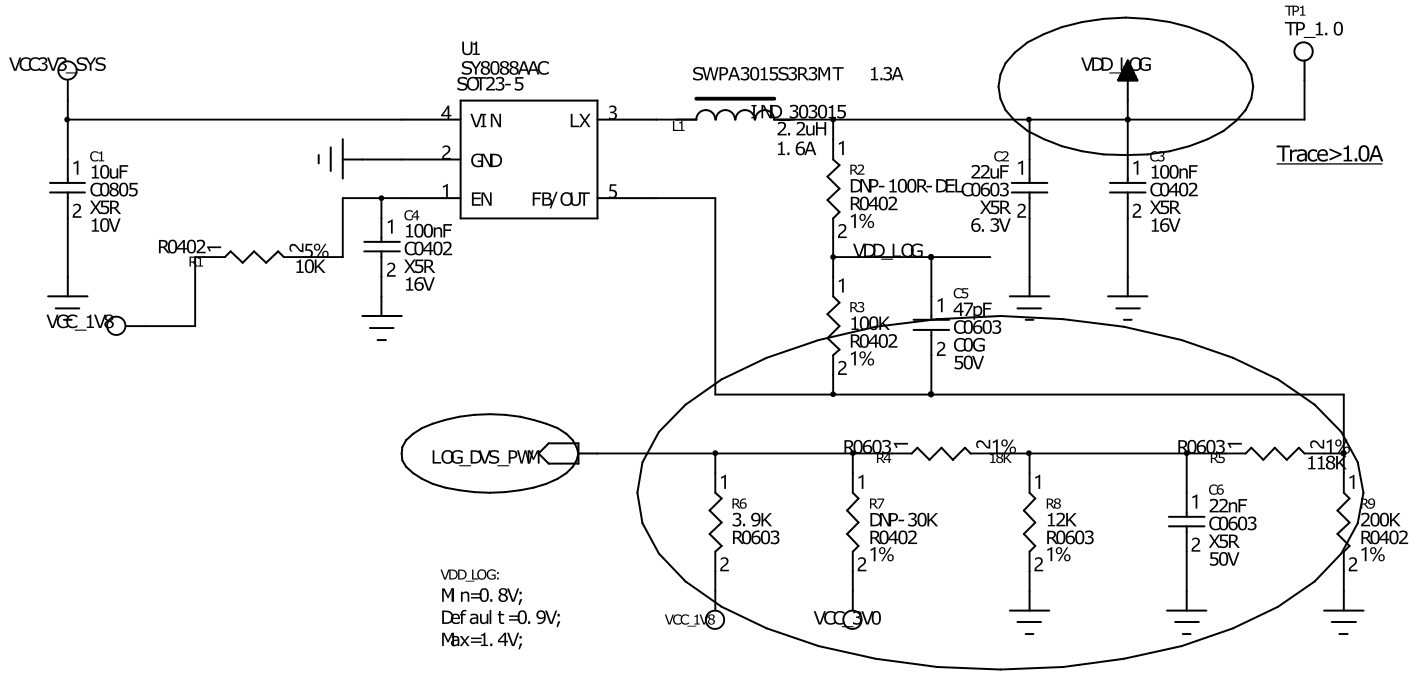5.2.1. 反馈网络设计
设流过 R5 进入 DC/DC 变换器的电流为 $I_{DAC}$, R5 左端的电压为 $V_{DAC}$, 则有
$I_{DAC}=\frac{V_{DAC}-V_{REF}}{R5}$
$V_{OUT}=V_{REF}(1+\frac{R3}{R9}) - I_{DAC}R3$
$V_{DAC}=V_{C}\frac{R8}{R8+R4}$
经整理可得
$V_{OUT}=V_{REF}(1+R3(\frac{R9+R5}{R9R5})) - \frac{R3}{R5}\frac{R8}{R8+R4}V_{C}$
$V_{OUT}=A - kV_{C}$
同时
$F_{PWM} > \frac{10}{R4C6}$
5.2.2. 反馈网络计算

假设 PWM 控制信号频率为 25kHz，占空比范围为 [0, 100%]
选用的变换器的 $V_{REF}=0.6V$, 要求控制电压范围为 $V_{C}=[0, 1.8V]$, 输出电压调整范围为 $V_{OUT}=[0.8, 1.4V]$
没接入 DVS 电路时, 要求输出电压为0.9V, 则 $R9=2R3$, 设 $R3=100K$, 则 $R9=200K$
当 $V_{C} = 0V$ 时, $V_{OUT} = 1.4V = A$, 可计算出 $R5=\frac{V_{REF}*R3*R9}{A*R9-V_{REF}*R9-V_{REF}*R3}=120K$
当 $V_{C} = 1.8V$ 时, $V_{OUT} = 0.8V$, 可计算出 $k=\frac{1}{3}$, 则 $\frac{R8}{R4}=\frac{2}{3}$, 则 $V_{DAC}=V_{C}\frac{2}{5}=0.72V$

设 $V_{C}$ 信号带载 ＜0.1mA，则 $R8 + R4 ＞ 1.8/0.1=18K$, $R4 ＞ 10.8K$

设 $R4=12K$, 则 $R8=8K$
设 $R4=15K$, 则 $R8=10K$ | √
设 $R4=18K$, 则 $R8=12K$ | √

为保证 $V_{C}$ 信号悬空时, 输入固定, 加入上拉电阻 R6, 当 $V_{C}$ 悬空时, $V_{OUT}≤=0.9V$, 可计算出 $R6≤=2R8-R4=6K$, 可取 $R6=3.9K$
假设控制频率为 $F_{PWM}=25kHz$, 则 $C1≤=22nF$, 可取 $C1=22nF$

5.2.3. 驱动配置修改

VC/LOG_DVS_PWM 接入 RK3399 GPIO，工作于 PWM 模式


vdd_log: vdd-log {
compatible = "pwm-regulator";
pwms = <&pwm2 0 25000 1>;
regulator-name = "vdd_log";
regulator-min-microvolt = <800000>;
regulator-max-microvolt = <1400000>;
regulator-always-on;
regulator-boot-on;

/* for rockchip boot on */
rockchip,pwm_id = <2>;
rockchip,pwm_voltage = <900000>;
};




展开全文• 针对复杂地质条件所造成的露天矿煤层突然急剧下降,形成洪峰剥采比,传统的超前剥离方法无法进行有效剥采调节的情况,运用露天采矿学的基本原理,提出了一种新的剥采比调节方法——双坑动态剥采调节方法,并详细分析了双...
• 【电路原理】傻瓜175是大功率模块，只有五只引脚，内部输出对管采用互补的大功率场效应管，外围不用任何元件。它输出功率大、线性好、频响宽、失真小，特别是其有对大动态信号响应迅速的特点，因而在中初级音响制作...
• 研究了人眼在近视力状态下自动调节产生的动态像差。采用哈特曼夏克(Hartmann-Shack, H-S)原理研制了一套可诱导人眼屈光调节,并能测量不同调节状态下人眼像差的测量仪。诱导原理为用视标在人眼近距离范围内移动以诱发...
• 在过程控制中，按偏差的比例(P)、积分(I)和微分(D)进行控制的PID控制器(亦称PID调节器)是应用最为广泛的一种自动控制器。它具有原理简单，易于实现，...PID调节规律是连续系统动态品质校正的一种有效方法，它的参数...
• 应用离散事件系统（DES）框架中的优化原理，建立数学模型，得到了能量消耗的目标函数，为了最小化目标函数，利用线性规划方法进行求解，得到一种可扩展的低复杂度算法来进行动态电压调节（DVS）。并对算法进行了模型...
• 为了实现嵌入式实时操作系统对DVS的支持，本文在分析软硬件两方面节能原理的基础上，提出了一个在实际应用中实现DVS的通用模型。该模型在μC/OSII上得到了具体的实现。
• 本文主要讲述cpufreq动态频率调节系统，详细讲到了相关设置流程及原理，最后以编译系统apk的形式实现cpu的定频处理功能cpufreq
• 1． 对温箱(即图中炉子部分）系统采用实验法--阶跃响应法建模，并提出合理的建模方法，建立该装置的数学模型； 2．学习MATLAB语言中有关自动控制系统仿真的内容；...4．要求系统输出动态性能满足无超调量，Ts≤10min
• 摘要 为了实现嵌入式实时操作系统对DVS的支持，本文在分析软硬件两方面节能原理的基础上，提出了一个在实际应用中实现DVS的通用模型。该模型在μC/OSII上得到了具体的实现。实现后的μC/OSII被移植到支持离散频率...
• 点击「iHydrostatics」，...想第一时间了解我们的最新动态？那就赶快加入液界联盟的会员吧！Webinar#名企大讲堂 主题研讨会液界的名企们，他们都已经来啦！你，在哪里？我们最专注、专业的小伙伴们可都在这里等你啦~...助力泵嗡嗡响解决方法
• 30 光照强度模块测量周围的光照强度，给单片机串口发送一个任意字符串就可以触发单片机采集一个光照强度值，打印到电脑的串口调试助手，同时单片机的数码管也显示光照强度值，由于数码管显示是动态显示的，所以数据...unity shader unity3d opengl
• 1、发射刺激信号人体的机能调节是受到一定的信号调节的，尤其是身体会存在反馈与负反馈的机制，让我们的身体处于一种动态平衡的状态。有的睡眠仪器可以发射出刺激的信号，从而让我们的大脑接受指令，做出相...
• CPU降频实现原理与试验数据 1. CPU降频实现原理 CPU降频主要是通过控制CPU的工作...1，performance ：顾名思义只注重效率，将CPU频率固定工作在其支持的最高运行频率上，而不动态调节。 2，powersave：将CPU频率设置
• 将改进后的溢流装置应用于生产实践,结果表明:改进后的调节装置操作简单,调节精度较高,且能够达到溢流动态调节的目的;磁选尾矿带介量和吨煤介质消耗量均降低,减少了矿山投入成本,极大地提高了矿山经济效益。
• 该控制回路的性能影响电源的关键性能参数，包括线路和负载调节，稳定性和动态响应。 工程师可以通过测量控制回路的频率响应来量化控制回路的性能。电压调节器可在很宽的频率范围内工作。然后可以通过将补偿器...
• 在酒泉风电基地现场测试了3种动态无功补偿装置的动态调节范围、动态响应时间及有功损耗,并根据测试结果进行了对比分析,提出了在同一升压站内安装不同类型的动态无功补偿装置,以利于充分发挥各自优势的建议。
• 一、原理　1、首先是电流环，此环完全在伺服驱动器内部进行，通过霍尔装置检测驱动器给电机的各相的输出电流，负反馈给电流的设定进行PID调节，从而达到输出电流尽量接近等于设定电流，电流环就是控制电机转矩的，...
• 自动控制原理 MATLAB分析和设计 仿真实验报告 第三章 线性系统的时域分析法 1 教材 P136.3-5 系统进行动态性能仿真 并和忽略闭环零点的系统动 态性能进行比较分析仿真结果 (1) 原系统的动态性能 SIMULINK 仿真图 ...
• 它可快速改变其发出的无功，具有较强的无功调节能力，可为电力系统提供动态无功电源、调节系统电压
• 针对传统的自动电压调节器测试方法存在测试项目较少、安全性差、建设成本高、设备参数差异大等缺陷,提出了一种基于LabWindows/CVI的自动电压调节器通用测试系统的设计方案,并介绍了系统的构成和原理。该系统实现了...
• 介绍了PMAC运动控制器中PID的控制原理，并对基于其上的数控试验台的PID参数调节进行了说明。利用PMAC自带的PID程序，通过对阶跃信号和抛物线信号响应图像的分析，整定了系统参数，使试验台获得良好的动态特性。
• 本文分析了永磁同步伺服系统的控制原理，对采用三环控制结构的伺服系统的控制器参数整定进行设计分析。传统伺服控制中应用最多的是PID控制，而调速系统要求以动态稳定和稳态精度为主，所以电流环...
• 通过对故障暂态阶段(换流器未响应阶段、换流器动态调节阶段)及故障稳态阶段的电流故障分量特征进行分析，得出故障全过程中整流和逆变侧故障分量差值在区内故障时满足正极性特征而区外故障时不满足的结论，建立面向...
• 了解定时器/计数器的工作原理。 掌握定时器/计数器的寄存器配置方法。 掌握定时器的使用方法。 结合按键检测和动态扫描显示实验，设计一个时钟程序。 要求： 使用8位数码管显示时、分、秒，之间用减号间隔...